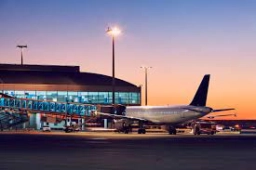# Airplane 81200

Two points at a distance of 1840 km. An airplane departs from A at 7:00 a.m. and flies at an average speed of 50 km/h faster than another airplane departs from B at 8:00 a.m. At what distance from A do they meet at 9:00?

x =  1260 km

### Step-by-step explanation:Did you find an error or inaccuracy? Feel free to write us. Thank you!

Tips for related online calculators
Do you have a linear equation or system of equations and looking for its solution? Or do you have a quadratic equation?
Do you want to convert velocity (speed) units?
Do you want to convert time units like minutes to seconds?